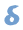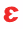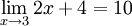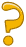# Limits (Formal Definition)

## Approaching ...

Sometimes we can't work something out directly ... but we can see what it should be as we get closer and closer!

### Example:

(x2 − 1) (x − 1)

Let's work it out for x=1:

(12 − 1) (1 − 1) = (1 − 1) (1 − 1) = 0 0

Now 0/0 is a difficulty! We don't really know the value of 0/0 (it is "indeterminate"), so we need another way of answering this.

So instead of trying to work it out for x=1 let's try approaching it closer and closer:

### Example Continued:

 x (x2 − 1) (x − 1) 0.5 1.50000 0.9 1.90000 0.99 1.99000 0.999 1.99900 0.9999 1.99990 0.99999 1.99999 ... ...

Now we see that as x gets close to 1, then (x2−1) (x−1) gets close to 2

We are now faced with an interesting situation:

• When x=1 we don't know the answer (it is indeterminate)
• But we can see that it is going to be 2

We want to give the answer "2" but can't, so instead mathematicians say exactly what is going on by using the special word "limit"

The limit of (x2−1) (x−1) as x approaches 1 is 2

And it is written in symbols as:So it is a special way of saying, "ignoring what happens when we get there, but as we get closer and closer the answer gets closer and closer to 2"

 As a graph it looks like this: So, in truth, we cannot say what the value at x=1 is. But we can say that as we approach 1, the limit is 2.## More Formal

But instead of saying a limit equals some value because it looked like it was going to, we can have a more formal definition.

## From English to Mathematics

Let's say it in English first:

"f(x) gets close to some limit as x gets close to some value"

When we call the Limit "L", and the value that x gets close to "a" we can say

"f(x) gets close to L as x gets close to a"## Calculating "Close"

Now, what is a mathematical way of saying "close" ... could we subtract one value from the other?

Example 1: 4.01 − 4 = 0.01Example 2: 3.8 − 4 = −0.2Hmmm ... negatively close? That doesn't work ... we really need to say "I don't care about positive or negative, I just want to know how far" which is the absolute value.

"How Close" = |a−b|

Example 1: |4.01−4| = 0.01Example 2: |3.8−4| = 0.2And when |a−b| is small we know we are close, so we write:

"|f(x)−L| is small when |x−a| is small"

And this animation shows what happens with the function

f(x) = (x2−1) (x−1)

f(x) approaches L=2 as x approaches a=1,
so |f(x)−2| is small when |x−1| is small.

## Delta and Epsilon

But "small" is still English and not "Mathematical-ish".

Let's choose two values to be smaller than:that |x−a| must be smaller thanthat |f(x)−L| must be smaller than

(Note: Those two greek letters, δ is "delta" and ε is "epsilon", are often
used for this, leading to the phrase "delta-epsilon")

And we have:

 "|f(x)−L|<when |x−a|<"

That actually says it! So if you understand that you understand limits ...

... but to be absolutely precise we need to add these conditions:

 1) 2) 3) it is true for any>0exists, and is >0 x not equal to a means 0<|x−a|

And this is what we get:

 "for any>0, there is a>0 so that |f(x)−L|<when 0<|x−a|<"

That is the formal definition. It actually looks pretty scary, doesn't it!

But in essence it still says something simple: when x gets close to a then f(x) gets close to L.

## How to Use it in a Proof

To use this definition in a proof, we want to go

 From: To: 0<|x−a|<|f(x)−L|<This usually means finding a formula for(in terms of) that works.

How do we find such a formula?

Guess and Test!

That's right, we can:

1. Play around till we find a formula that might work
2. Test to see if that formula works.

## Example: Let's try to show thatUsing the letters we talked about above:

• The value that x approaches, "a", is 3
• The Limit "L" is 10

So we want to know:

 How do we go from: 0<|x−3|<to |(2x+4)−10|<### Step 1: Play around till you find a formula that might work

 Start with: |(2x+4)−10|<Simplify: |2x−6|<Move 2 outside: 2|x−3|<Move 2 across: |x−3|</2

So we can now guess that=/2 might work

### Step 2: Test to see if that formula works.

So, can we get from 0<|x−3|<to |(2x+4)−10|<... ?

Let's see ...

 Start with: 0<|x−3|<Replace: 0<|x−3|</2 Move 2 across: 0<2|x−3|<Move 2 inside: 0<|2x−6|<Replace "−6" with "+4−10" 0<|(2x+4)−10|<Yes! We can go from 0<|x−3|<to |(2x+4)−10|<by choosing=/2

DONE!

We have seen then that givenwe can find a, so it is true that:

"for any, there is aso that |f(x)−L|<when 0<|x−a|<"

And we have proved that## Conclusion

That was a fairly simple proof, but it hopefully explains the strange "there is a ... " wording, and it does show you a good way of approaching these kind of proofs.